# Difference between revisions of "Largest prime power divisor"

This article defines an arithmetic function or number-theoretic function: a function from the natural numbers to a ring (usually, the ring of integers, rational numbers, real numbers, or complex numbers).
View a complete list of arithmetic functions

## Definition

Letbe a natural number. The largest prime power divisor of, sometimes denoted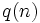and sometimes denoted, is defined as the largest prime power that divides.

## Behavior

The ID of the sequence in the Online Encyclopedia of Integer Sequences is A034699

### Upper bound

The value of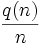is largest whenitself is a prime power, namely, it isfor these values of. Since there are infinitely many primes, we have:.

### Lower bound

Further information: Largest prime power divisor has logarithmic lower bound

The largest prime power divisor ofis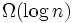. In fact, we have: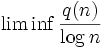is finite and greater than zero.

Thus, we have:.

### Asymptotic fraction

Further information: Fractional distribution of largest prime power divisor

The value ofis almost uniformly distributed in the interval.

## Relation with other arithmetic functions

• Prime divisor count function: This is the total number of prime divisors of, and is denoted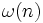. We have the following relation:.